# 基于K近邻的电影推荐与分级预测

### 推荐系统的类型

1. 协同过滤

2. 基于内容的筛选

3. 混合推荐系统

#### 协同过滤

• 用户-用户协同过滤：尝试搜索相似的客户，并根据他/她的相似选择提供产品。
• 项-项协同过滤：它与前面的算法非常相似，但是我们没有找到一个看起来很相似的客户，而是尝试查找相似的项。一旦我们有了商品外观相似矩阵，我们就可以很容易地向从商店购买商品的顾客推荐相似的商品。
• 其他算法：还有其他方法，如市场篮子分析，它通过寻找交易中经常出现的项目组合来工作。#### 混合推荐系统Netflix是使用混合推荐系统的一个很好的例子。该网站通过比较相似用户的观看和搜索习惯(即协作过滤)以及提供与用户评价较高的电影具有相同特征的电影(基于内容的过滤)来提出建议。

#### TMDb-电影数据库• tmdb_5000_movies.包含分数、标题、发布日期、流派等信息。
• tmdb_5000_credits.csv:包含每部电影的演员和剧组信息。

### Python实现

#### 步骤1-导入数据集

movies = pd.read_csv('../input/tmdb-movie-metadata/tmdb_5000_movies.csv')
credits = pd.read_csv('../input/tmdb-movie-metadata/tmdb_5000_credits.csv')

#### 步骤2-数据探索和清理

movies.head()movies.describe()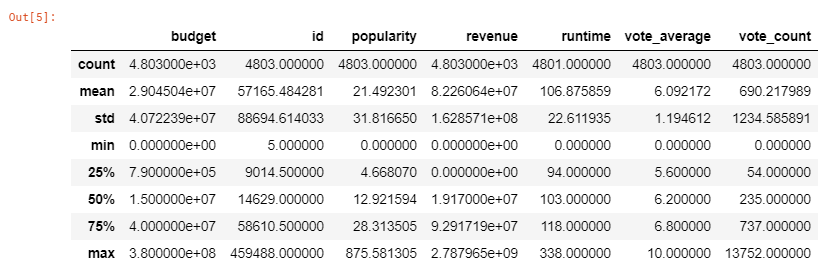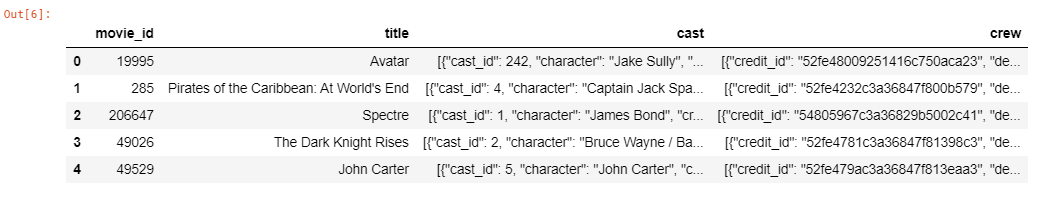JSON格式类似于嵌入到字符串中的dictionary(key:value)对。一般来说，解析数据在计算上是昂贵和耗时的。幸运的是，这个数据集没有那么复杂的结构。列之间的一个基本相似之处是它们有一个name键，它包含我们需要收集的值。最简单的方法是解析JSON并检查每行的name键。找到name键后，将其值存储到一个列表中，并用list替换JSON。

# 将genres列从json更改为string
for index,i in zip(movies.index,movies['genres']):
list1 = []
for j in range(len(i)):
list1.append((i[j]['name'])) # “name”包含流派的名称
movies.loc[index,'genres'] = str(list1)#### 步骤3-合并2个CSV文件

movies = movies.merge(credits, left_on='id', right_on='movie_id', how='left')
movies = movies[['id', 'original_title', 'genres', 'cast', 'vote_average', 'director', 'keywords']]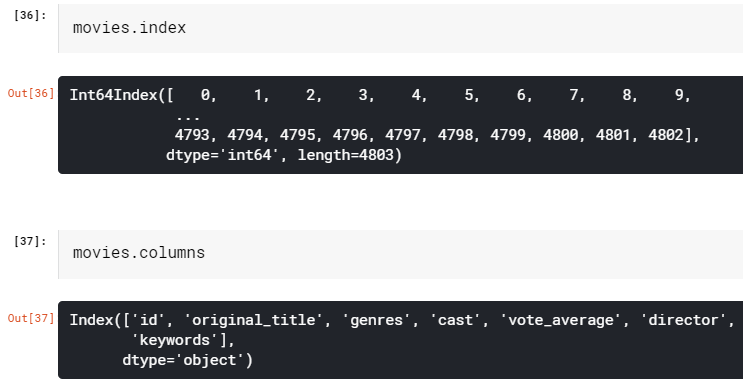#### 第4步-使用“Genres”列

movies['genres'] = movies['genres'].str.strip('[]').str.replace(' ','').str.replace("'",'')
movies['genres'] = movies['genres'].str.split(',')

plt.subplots(figsize=(12,10))
list1 = []
for i in movies['genres']:
list1.extend(i)
ax = pd.Series(list1).value_counts()[:10].sort_values(ascending=True).plot.barh(width=0.9,color=sns.color_palette('hls',10))
for i, v in enumerate(pd.Series(list1).value_counts()[:10].sort_values(ascending=True).values):
ax.text(.8, i, v,fontsize=12,color='white',weight='bold')
plt.title('Top Genres')
plt.show()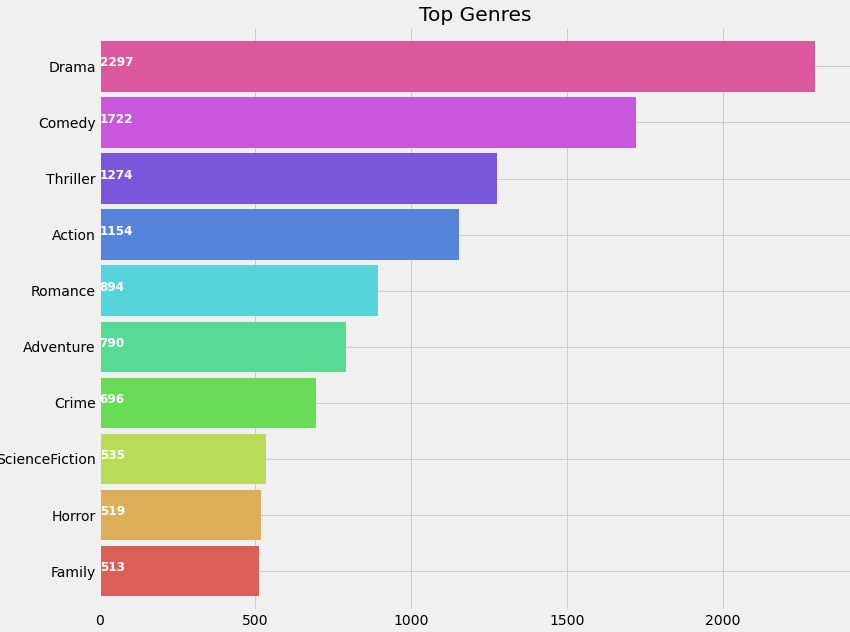genreList = []
for index, row in movies.iterrows():
genres = row["genres"]

for genre in genres:
if genre not in genreList:
genreList.append(genre)
genreList[:10] # 现在我们有了一个流派的列表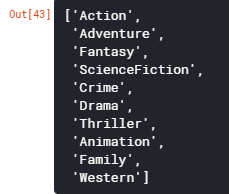#### one-hot编码

“genreList”将保存所有流派。但是我们如何才能知道每部电影所属的类型呢。现在有些电影是“动作片”，有些是“动作片，冒险片”，等等。我们需要根据电影类型对电影进行分类。

def binary(genre_list):
binaryList = []

for genre in genreList:
if genre in genre_list:
binaryList.append(1)
else:
binaryList.append(0)

return binaryList

movies['genres_bin'] = movies['genres'].apply(lambda x: binary(x))
movies['genres_bin'].head()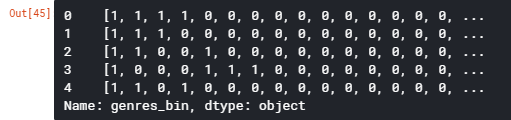#### 步骤5-使用Cast列

plt.subplots(figsize=(12,10))
list1=[]
for i in movies['cast']:
list1.extend(i)
ax=pd.Series(list1).value_counts()[:15].sort_values(ascending=True).plot.barh(width=0.9,color=sns.color_palette('muted',40))
for i, v in enumerate(pd.Series(list1).value_counts()[:15].sort_values(ascending=True).values):
ax.text(.8, i, v,fontsize=10,color='white',weight='bold')
plt.title('Actors with highest appearance')
plt.show()for i,j in zip(movies['cast'],movies.index):
list2 = []
list2 = i[:4]
movies.loc[j,'cast'] = str(list2)
movies['cast'] = movies['cast'].str.strip('[]').str.replace(' ','').str.replace("'",'')
movies['cast'] = movies['cast'].str.split(',')
for i,j in zip(movies['cast'],movies.index):
list2 = []
list2 = i
list2.sort()
movies.loc[j,'cast'] = str(list2)
movies['cast']=movies['cast'].str.strip('[]').str.replace(' ','').str.replace("'",'')castList = []
for index, row in movies.iterrows():
cast = row["cast"]

for i in cast:
if i not in castList:
castList.append(i)movies[‘cast_bin’] = movies[‘cast’].apply(lambda x: binary(x))
movies[‘cast_bin’].head()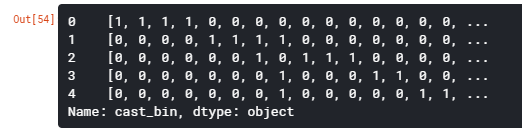#### 第6步-使用“Directors ”列

def xstr(s):
if s is None:
return ''
return str(s)
movies['director'] = movies['director'].apply(xstr)plt.subplots(figsize=(12,10))
ax = movies[movies['director']!=''].director.value_counts()[:10].sort_values(ascending=True).plot.barh(width=0.9,color=sns.color_palette('muted',40))
for i, v in enumerate(movies[movies['director']!=''].director.value_counts()[:10].sort_values(ascending=True).values):
ax.text(.5, i, v,fontsize=12,color='white',weight='bold')
plt.title('Directors with highest movies')
plt.show()directorList=[]
for i in movies['director']:
if i not in directorList:
directorList.append(i)movies['director_bin'] = movies['director'].apply(lambda x: binary(x))
movies.head()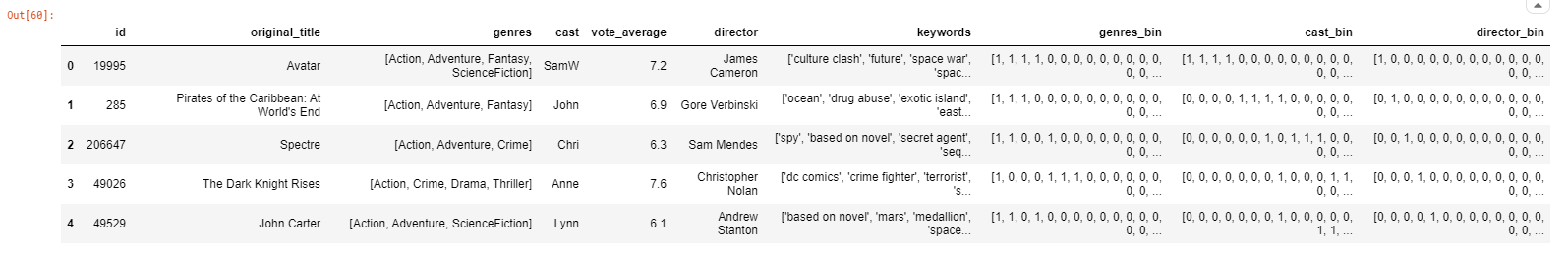#### 步骤7-使用Keywords列

from wordcloud import WordCloud, STOPWORDS
import nltk
from nltk.corpus import stopwordsplt.subplots(figsize=(12,12))
stop_words = set(stopwords.words('english'))
stop_words.update(',',';','!','?','.','(',')','\$','#','+',':','...',' ','')words=movies['keywords'].dropna().apply(nltk.word_tokenize)
word=[]
for i in words:
word.extend(i)
word=pd.Series(word)
word=([i for i in word.str.lower() if i not in stop_words])
wc = WordCloud(background_color="black", max_words=2000, stopwords=STOPWORDS, max_font_size= 60,width=1000,height=1000)
wc.generate(" ".join(word))
plt.imshow(wc)
plt.axis('off')
fig=plt.gcf()
fig.set_size_inches(10,10)
plt.show()movies['keywords'] = movies['keywords'].str.strip('[]').str.replace(' ','').str.replace("'",'').str.replace('"','')
movies['keywords'] = movies['keywords'].str.split(',')
for i,j in zip(movies['keywords'],movies.index):
list2 = []
list2 = i
movies.loc[j,'keywords'] = str(list2)
movies['keywords'] = movies['keywords'].str.strip('[]').str.replace(' ','').str.replace("'",'')
movies['keywords'] = movies['keywords'].str.split(',')
for i,j in zip(movies['keywords'],movies.index):
list2 = []
list2 = i
list2.sort()
movies.loc[j,'keywords'] = str(list2)
movies['keywords'] = movies['keywords'].str.strip('[]').str.replace(' ','').str.replace("'",'')
movies['keywords'] = movies['keywords'].str.split(',')words_list = []
for index, row in movies.iterrows():
genres = row["keywords"]

for genre in genres:
if genre not in words_list:
words_list.append(genre)movies['words_bin'] = movies['keywords'].apply(lambda x: binary(x))
movies = movies[(movies['vote_average']!=0)] #删除得分为0且没有drector名称的电影
movies = movies[movies['director']!='']

#### 步骤8-电影之间的相似性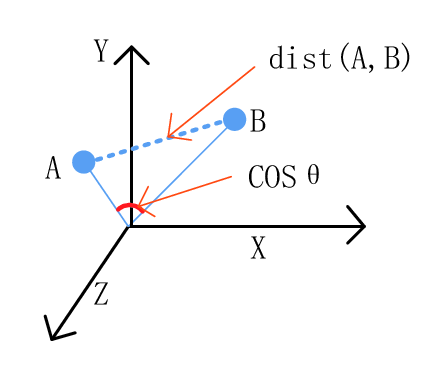from scipy import spatialdef Similarity(movieId1, movieId2):
a = movies.iloc[movieId1]
b = movies.iloc[movieId2]

genresA = a['genres_bin']
genresB = b['genres_bin']

genreDistance = spatial.distance.cosine(genresA, genresB)

scoreA = a['cast_bin']
scoreB = b['cast_bin']
scoreDistance = spatial.distance.cosine(scoreA, scoreB)

directA = a['director_bin']
directB = b['director_bin']
directDistance = spatial.distance.cosine(directA, directB)

wordsA = a['words_bin']
wordsB = b['words_bin']
wordsDistance = spatial.distance.cosine(directA, directB)
return genreDistance + directDistance + scoreDistance + wordsDistance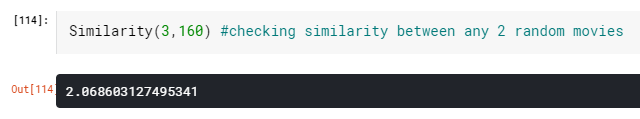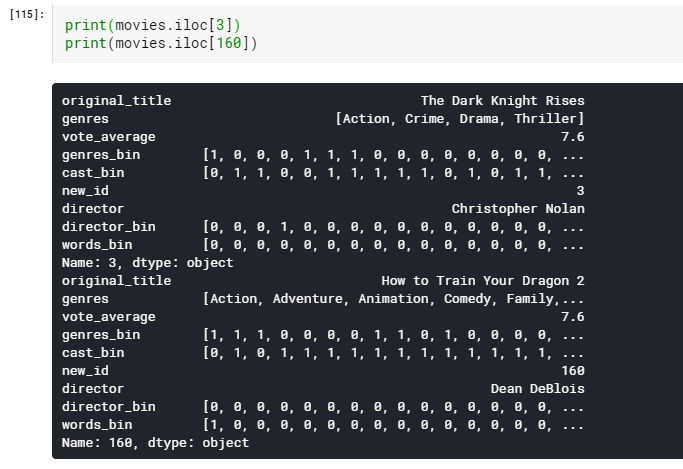#### 步骤9-分数预测器

import operatordef predict_score():
name = input('Enter a movie title: ')
new_movie = movies[movies['original_title'].str.contains(name)].iloc.to_frame().T
print('Selected Movie: ',new_movie.original_title.values)
def getNeighbors(baseMovie, K):
distances = []

for index, movie in movies.iterrows():
if movie['new_id'] != baseMovie['new_id'].values:
dist = Similarity(baseMovie['new_id'].values, movie['new_id'])
distances.append((movie['new_id'], dist))

distances.sort(key=operator.itemgetter(1))
neighbors = []

for x in range(K):
neighbors.append(distances[x])
return neighbors

K = 10
avgRating = 0
neighbors = getNeighbors(new_movie, K)print('\nRecommended Movies: \n')
for neighbor in neighbors:
avgRating = avgRating+movies.iloc[neighbor]
print( movies.iloc[neighbor]+" | Genres: "+str(movies.iloc[neighbor]).strip('[]').replace(' ','')+" | Rating: "+str(movies.iloc[neighbor]))

print('\n')
avgRating = avgRating/K
print('The predicted rating for %s is: %f' %(new_movie['original_title'].values,avgRating))
print('The actual rating for %s is %f' %(new_movie['original_title'].values,new_movie['vote_average']))

predict_score()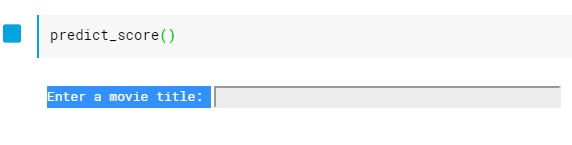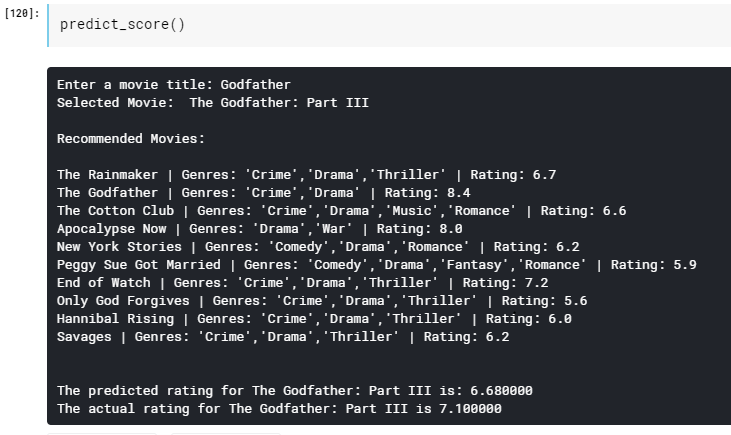#### 进一步阅读

http://panchuang.net/

sklearn机器学习中文官方文档：
http://sklearn123.com/

http://docs.panchuang.net/